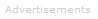# Definition of Coulombic Attraction

What is Coulombic Attraction?

Coulombic attraction is the force of attraction between positive and negative charges.

It is easy to calculate the force between two charged particles using Coulomb's law. If the charges on the particles have opposite signs, the force will be one of attraction. If the charges on the particles have the same signs, the force will be one of repulsion.

F = kq1q2 / r2

Where:

F is force, in newtons

k is Coulomb's constant. k's value depends on the medium around the charged objects. In air, k is approximately 9.0 x 109 N m2 C-2.

q1 is the charge on the first particle, measured in coulombs.

q2 is the charge on the second particle, measured in coulombs.

r is the distance between the particles, in meters.Search the Dictionary for More Terms